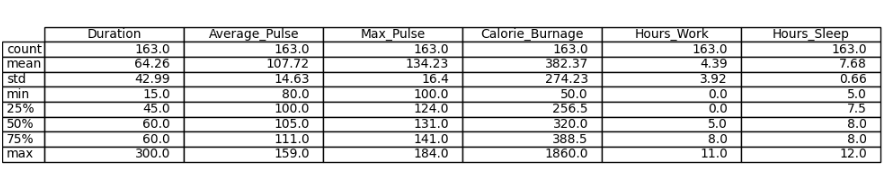# Data Science - Intro to Statistics

## Introduction to Statistics

Statistics is the science of analyzing data.

When we have created a model for prediction, we must assess the prediction's reliability.

After all, what is a prediction worth, if we cannot rely on it?

## Descriptive Statistics

We will first cover some basic descriptive statistics.

Descriptive statistics summarizes important features of a data set such as:

• Count
• Sum
• Standard Deviation
• Percentile
• Average
• Etc..

It is a good starting point to become familiar with the data.

We can use the `describe()` function in Python to summarize the data:

### Example

print (full_health_data.describe())
Try it Yourself »

Output:Do you see anything interesting here?